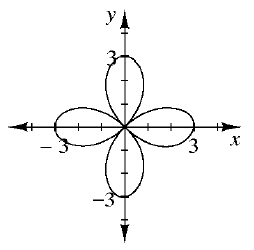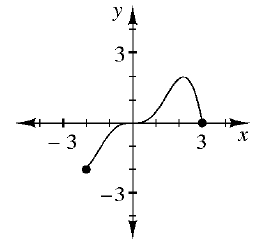### Home > AC > Chapter 12 > Lesson 12.1.2 > Problem12-19

12-19.

Examine the graphs of each relation below. Decide if each is a function. Then describe the domain and range of each.

1.Is there more than one output value for a single input value? If so, it is not a function.

Determine the possible $x$-values to find the domain and the possible $y$-values to find the range.

It is not a function.
R: $-3\le x\le3$

1.Is there more than one output value for a single input value? If so, it is not a function.

Determine the possible $x$-values to find the domain and the possible $y$-values to find the range.

It is a function.
D: $-2\le x\le3$